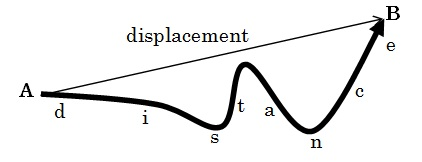# Difference Between Average Speed and Average Velocity

## Main Difference – Average Speed vs. Average Velocity

Average speed and average velocity are both measurements of rate of change of position in a body. The main difference between average speed and average velocity is that the average speed is calculated as the total distance traveled by a body divided by the time taken, while average velocity is the displacement divided by the time taken. Both quantities have units ms-1. For an object moving in a straight line, the magnitude of average velocity is equal to the body’s average speed.

## What is Average Speed

Average speed of a body is the total distance traveled by a body divided by the time taken. distance is a measurement of the total path length traveled by an object. Distance is a scalar quantity (it has no specific direction). Hence average speed is also a scalar quantity.

## What is Average Velocity

Average velocity is the displacement divided by the time taken. Displacement measures the shortest distance between the object’s initial position and its final position. It is a vector quantity with the direction of the vector being the direction from the starting point to the final point. Since displacement is a vector quantity, average velocity is also a vector quantity.

The diagram below shows the difference between distance and displacement:Difference Between Average Speed and Average Velocity – Displacement vs Distance

## Difference Between Average Speed and Average Velocity – An Example

An Olympic track has a circumference of 400 m. The World Record for women’s 400 m sprint was set in 1985 by Marita Koch of (what was then) East Germany. If her record was a time of 47.60 s, find,

a) her average speed

b) her average velocity

for her record-setting 400 m sprint.

a) Her average speed is simply the total distance (400 m) divided by the total time (47.60 s)$\mathrm{average\:speed=\frac{total\:distance}{time\:taken}=\frac{400\:m}{47.60\:s}=8.40\:m\:s^{-1}}$

b) 400 m include one complete round around the track. In the end, Marita needs to come back to the starting point to finish the race. This means that her displacement is 0.$\mathrm{average\:velocity=\frac{displacement}{time\:taken}=\frac{0\:m}{47.60\:s}=0\:m\:s^{-1}}$

## Difference Between Average Speed and Average Velocity

### Definition of Average Speed and Average Velocity

Average speed is the total distance traveled divided by the time. Speed takes into account the total path length traveled.

Average velocity is the displacement divided by the time. Velocity only takes into account the starting and ending points of an object’s path.

### Direction

Average speed has no specific direction. It is a scalar quantity.

Average velocity has a specified direction. It is a vector quantity.

###### “ Marita Koch at the 1984 East German Championships in Athletics in Erfurt, Thuringia, Germany. She was the winner in the 400-meter race for women.” by Kluge, Wolfgang (German Federal Archives ) [CC BY-SA 3.0 DE], via Wikimedia Commons